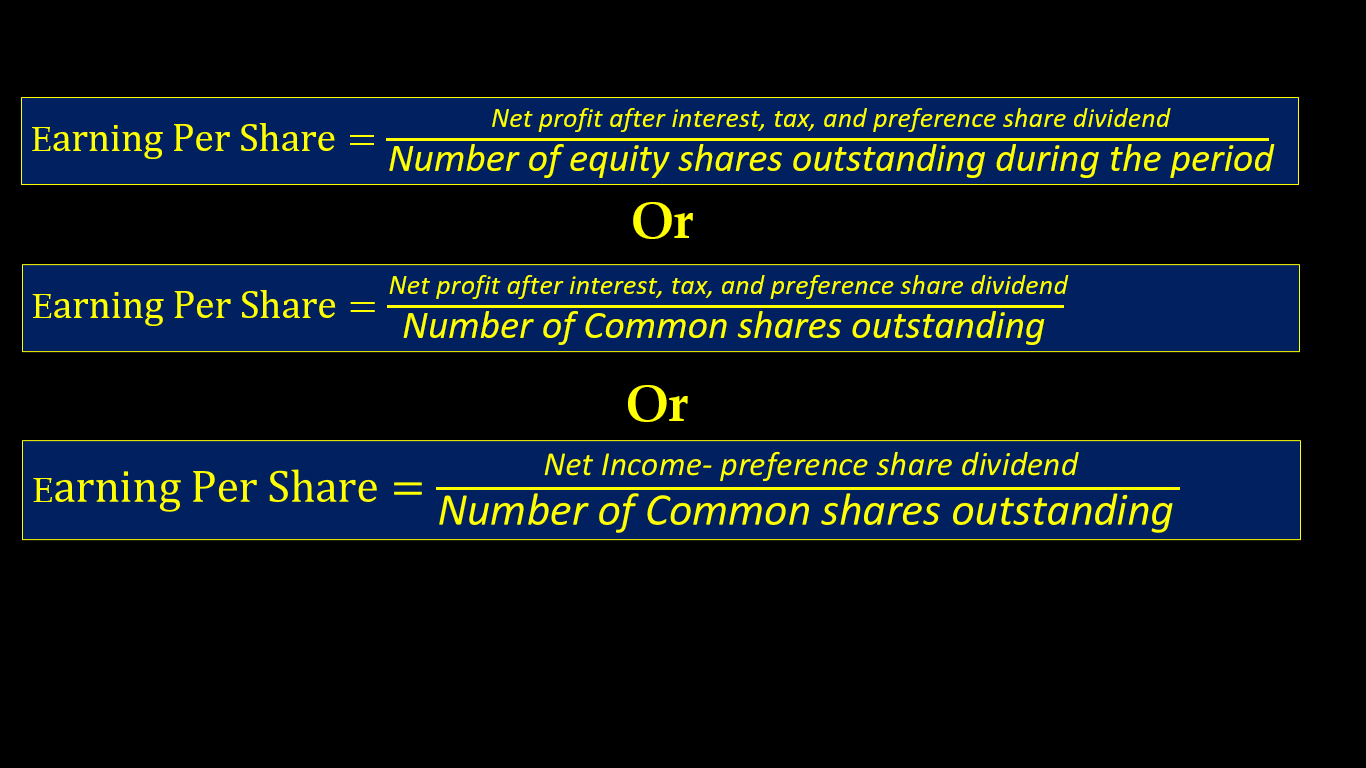# Earnings Per Share (EPS)

## Earnings Per Share (EPS):

Earning per share(EPS) shows the relationship between net profit after interest, tax and preference share dividend, and number of equity shares.
KEY POINTS:
*
The Earning per share(EPS) is calculated by dividing the net profit after interest, tax, and preference share dividend by the number of equity shares outstanding during the period.
*  The Earning per share ratio shows the profitability of the company on per share basis.
* Earnings per share or EPS is an important financial measure, which indicates the profitability of a company.
* Earning per share(EPS) shows the relationship between profit available for equity shareholders, and number of equity shares.

## The formula for Earnings Per Share (EPS):## Examples For Earnings Per Share (EPS)

Example 1.

Calculate earning per share from the following information:
Net profit after interest tax and preference share dividend ₹19,20,000.
Equity share capital ₹20,00,000(Each share ₹10)
10 % Preference share capital ₹5,00,000.

Solution:Earning per share=₹19,20,000/2,00,000
Earning per share=₹9.6per share

Example 2.

Calculate earning per share from the following information:
Net profit after interest and tax but before preference share dividend ₹12,50,000.
Equity share capital ₹10,00,000(Each share ₹10)
10% Preference share capital ₹5,00,000.

Solution:Net profit after interest and tax but before preference share dividend ₹12,50,000
Preference share dividend=₹5,00,000×10/100
Preference share dividend=₹50,000

Net profit after interest, tax, and preference share dividend=₹12,50,000-₹50,000
Net profit after interest, tax, and preference share dividend=₹12,00,000

Earning per share=₹12,00,000/1,00,000
Earning per share=₹12per share

Example 3.

Calculate earning per share from the following information:
Net profit before interest and tax preference share dividend ₹13,70,000.
Equity share capital ₹10,00,000(Each share ₹10)
10% Preference share capital ₹7,00,000.
15% Debentures ₹8,00,000.
Rate of tax 40%

Solution:Net profit before interest ,tax and preference share dividend ₹13,70,000
Interest on Debentures ₹8,00,000×15/100=₹1,20,000
Net profit afte interest= ₹13,70,000-₹1,20,000=₹12,00,000
Amount of tax=₹12,00,000×40/100=₹4,80,000
Preference share dividend=₹7,00,000×10/100
Preference share dividend=₹70,000

Net profit after interest, tax, and preference share dividend=₹13,70,000-₹1,20,000-4,80,000-70,000
Earning per share=₹7,00,000/1,00,000
Earning per share=₹7per share

Example 4.

Calculate earning per share from the following information:
Net profit before interest and tax preference share dividend ₹16,60,000.
Equity share capital ₹10,00,000(Each share ₹10)
10% Preference share capital ₹5,00,000.
12% Debentures ₹8,00,000.
8% Second Debentures ₹8,00,000.
Rate of tax 40%

Solution:Net profit before interest ,tax and preference share dividend ₹16,60,000
Interest on Debentures ₹8,00,000×12/100=₹96,000
Interest on Second Debentures ₹8,00,000×8/100=₹64,000
Net profit after interest= ₹16,60,000-₹96,000-₹64,000=₹15,00,000
Amount of tax=₹15,00,000×40/100=₹6,00,000
Preference share dividend=₹5,00,000×10/100
Preference share dividend=₹50,000

Net profit after interest, tax, and preference share dividend=₹16,60,000-₹1,60,000-6,00,000-50,000
Earning per share=₹9,00,000/1,00,000
Earning per share=₹9per share

Liquid Ratio Or Quick Ratio Or Acid Test Ratio

Operating Ratio

Example 5.

Calculate earning per share from the following information:
Equity share capital ₹10,00,000(In share ₹10 each)
10% Preference share capital ₹10,00,000. (In share ₹100 each)
12% Debentures ₹8,00,000.
Rate of tax 40%
Net profit after tax ₹15,00,000
Solution:Net profit after tax ₹15,00,000
Preference share dividend=₹10,00,000×10/100
Preference share dividend=₹1,00,000

Net profit after interest, tax, and preference share dividend=₹15,00,000-₹1,00,000= ₹14,00,000
Earning per share=₹14,00,000/1,00,000
Earning per share=₹14 per share

Example 6.

Calculate earning per share from the following information:
Equity share capital ₹8,00,000(In share ₹10 each)
10% Preference share capital ₹15,00,000. (In share ₹100 each)
12% Debentures ₹8,00,000.
provision for tax ₹2,00,000.
Net profit after tax ₹13,50,000.
Solution:Net profit after tax ₹13,50,000
Preference share dividend=₹15,00,000×10/100
Preference share dividend=₹1,50,000

Net profit after interest, tax, and preference share dividend=₹13,50,000-₹1,50,000= ₹12,00,000
Earning per share=₹12,00,000/80,000
Earning per share=₹15 per share

Example 7.

Calculate earning per share from the following information:
Net profit before interest and tax preference share dividend ₹17,00,000.
Equity share capital ₹10,00,000(Each share ₹10)
10% Preference share capital ₹25,00,000.
12% Debentures ₹10,00,000.
10% Second Debentures ₹8,00,000.
Rate of tax 40%

Solution:Net profit before interest ,tax and preference share dividend ₹17,00,000
Interest on Debentures ₹10,00,000×12/100=₹1,20,000
Interest on Second Debentures ₹8,00,000×10/100=₹80,000
Net profit after interest= ₹17,00,000-₹2,00,000=₹15,00,000
Amount of tax=₹15,00,000×40/100=₹6,00,000
Preference share dividend=₹25,00,000×10/100
Preference share dividend=₹2,50,000

Net profit after interest, tax, and preference share dividend=₹17,00,000-₹2,00,000-₹6,00,000-₹2,50,000=
Earning per share=₹6,50,000/1,00,000
Earning per share=₹6.5per share